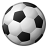# QlikView App Dev

Discussion Board for collaboration related to QlikView App Development.

Announcements
QlikWorld 2023, a live, in-person thrill ride. Save \$300 before February 6: REGISTER NOW!
cancel
Showing results for
Did you mean:Contributor II

## QV Variable in variable

Hi Everyone,

At the moment i'm trying to create a new variable which contains an existing variable.

The existing variable will generate a period of time , for example: 201901, 201902.

I want to check on the last 2 digits from the result of the existing variable, after I want to receive another period which I can hardcode. Result might be 201812 or 201912. So the current of previous year with '12 or 06' behind it.

This is my variable:

=if(right(max(=\$(vBTPlanperiodeCY)),2)='01' ,right(num( Year(AddMonths(Today(),-1)) ),2) & '12',
if(right(max(=\$(vBTPlanperiodeCY)),2)='02' ,right(num( Year(AddMonths(Today(),-1)) ),2) & '06'))

Always give the error: ')' expecting.

Anyone know which is the problem or how I can solve it?

Labels (1)
• ### Variables

2 RepliesCreator III

Try

=if(right(max(\$(vBTPlanperiodeCY)),2)='01' ,right(num( Year(AddMonths(Today(),-1)) ),2) & '12',
if(right(max(\$(vBTPlanperiodeCY)),2)='02' ,right(num( Year(AddMonths(Today(),-1)) ),2) & '06'))

Its not throwing an error, but im not sure if this is the solution. You can post some sample data if you still need some help.

Thanks!

ArpitContributor III

Hi Thomas,

You can use below expression.

=if(right(\$(vBTPlanperiodeCY),2)='01',
if(right(\$(vBTPlanperiodeCY),2)='02' ,right(num( Year(AddMonths(Today(),-1)) ),2) & '06'))

If vBTPlanperiodeCY=201902, then above exp is giving 1906 as an output.

if you want the output as 201906
then use below one.

=if(right(\$(vBTPlanperiodeCY),2)='01',